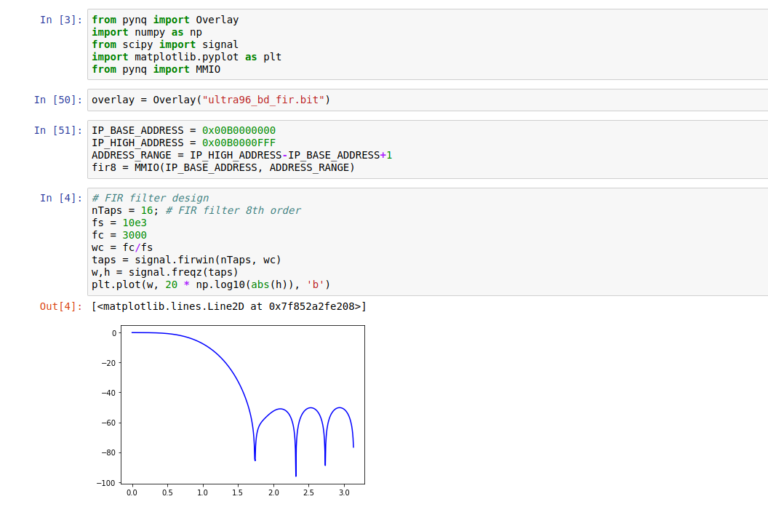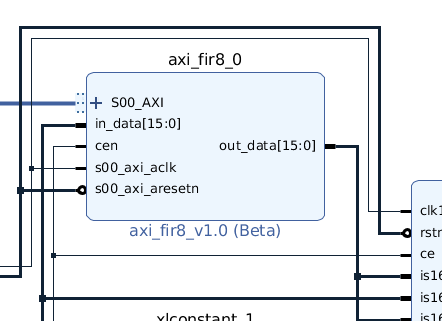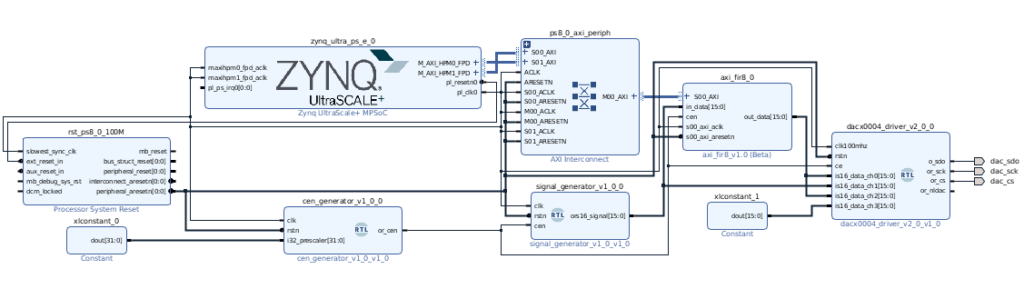We are in front of our customers, we have our design fully tested in our lab, all analog and digital filters works perfectly, but, in the customer installations there are an unknonw noise source. In this case, we must recompute digital filters, and spend time re sintesizing and implementing. Maybe this situation is a bit exaggerated, but there are a lot of situations where modify the characteristics of our filters in deployment phase may be very helpful.

Equations for compute the coefficients for digital filters, may be easy for low order FIR filters, or very complicated for several order IIR filters. Luckily, there are some tools that can kelp us to compute them. Most used tool for this purpose, surely is Matlab, but there are good open source alternatives like Octave or Python’s library Numpy and Scipy, and is the last one the way that I’ll show you in the next project using Pynq.

First of all, we need an IP with a filter, where the coefficients are sended through AXI. In 96analogxperience repository, you can find the IP axi_fir0_v1.0, that is a 8 order FIR filter with configurable coefficients through AXI interface. Input and output widths are configured when the IP is instantiated. Since the 96AnalogXperience works with 16 bits, we will use this width for input and output. INput and output signals, for this example will use Q15 format. For the coefficients, AXI provide us a efficient way to interchange parameter of 32 and 64 bits. IN this case, we will use parameters with 32 bits, this value is fixed inside the IP code, and we will configure the decimal at 31. Decimal width of parameters will depend of the paramaters, since if their values are in the range +-1, we can use only o bit for the integer part, but if the parameters have bigger values, the decimal part needs to be reconfigured.In addition to the AXI filter, we will need some modules. First, we need a clock enable generator for generate a one clock cycle signal at desired frequency,cen_generator_v1_0,v, and a module where we will generate the signal that we will filter, signal_generator_v1_0.v. This last module, read signal values from BRAM infered. Values in BRAM are generated with a python script named mem_generator. This script is written for a jupyter notebook.This design is designed to work in AVNET’s Ultra96 board, and my 96AnalogXperience. If you don’t know this mezzanine card, you can find the project on hackster.io. The Github repository includes a script for redraw the block design and configure all, but feel free to use the IP for modify or improve the design and, if you want, share it in the comments field. Below this lines, are the script for design the FIR filter, and configure the AXI IP through MMIO interface.

from pynq import Overlay
import numpy as np
from scipy import signal
import matplotlib.pyplot as plt
from pynq import MMIO

# Configure the overlay
overlay = Overlay("ultra96_bd_fir.bit")

# Define the IP addresses and definition

# FIR filter design
nTaps = 8; # FIR filter 8th order

fs = 10e3/2 # This value is corresponding with the Nyquist frequency
fc = 3000
wc = fc/fs
taps = signal.firwin(nTaps, wc)
w,h = signal.freqz(taps)
plt.plot(w, 20 * np.log10(abs(h)), 'b')

# Filter quantification
nBits = 32
tapsQ = []
for i in taps:
tapsQ.append(np.int(i*2**(nBits-1)))

# Write filter coefficients on IP
coeffIndex = 0
for j in tapsQ:
fir8.write(coeffIndex,j)
coeffIndex = coeffIndex+4